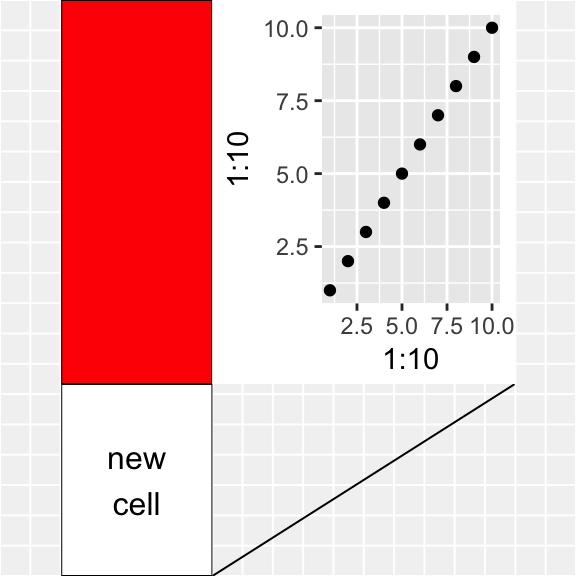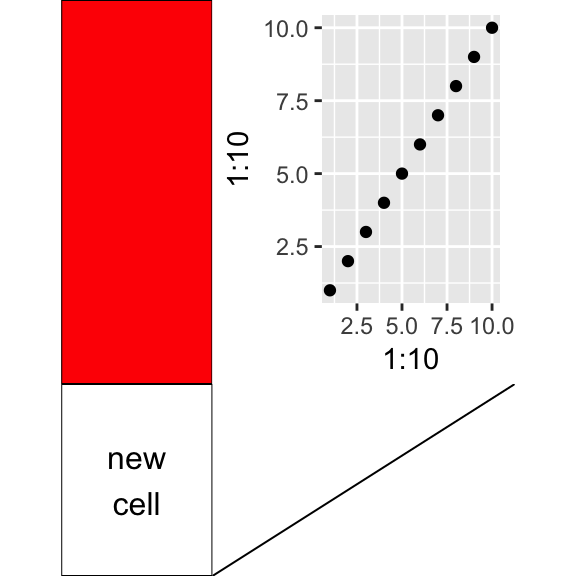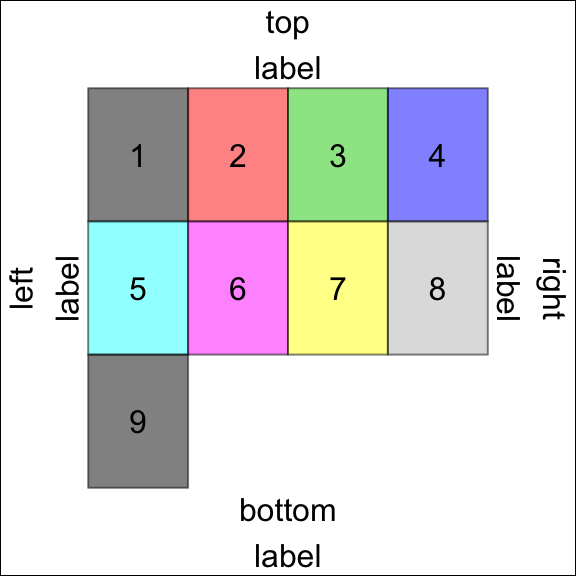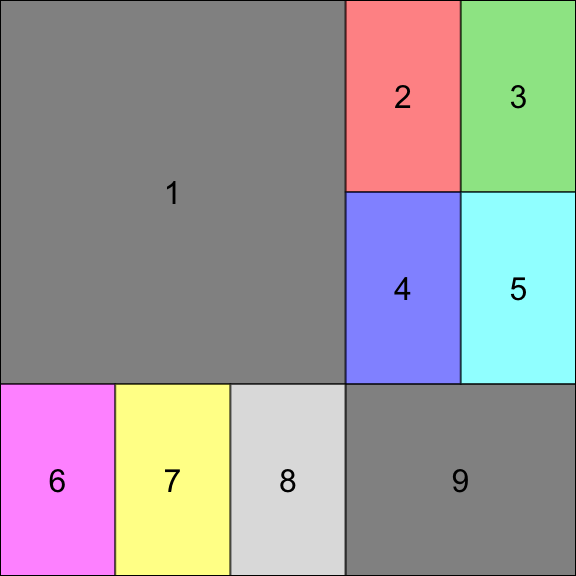(Unofficial) overview of gtable

2017-09-09

The R package gtable is designed to help construct and manipulate layouts containing graphical elements. The standard grid package in R provides low-level functions to define viewports, and place graphical elements (grobs) at specific locations within the device window. gtable builds upon these functions and provides a higher-level interface, where one can e.g. merge two layouts, add columns, rows, insert graphical elements in a given cell, and change the display order, among other things.

The gtable package is used internally by ggplot2, and can therefore be used to modify the layout of such plots.

Constructing a gtable

A gtable object can be constructed in a variety of ways,

• Empty table
gtable(unit(1:3, c("cm")), unit(5, "cm"))

This is an empty table with 3 rows and one column. gtable_col and gtable_row provide a simplified interface for 1 column or 1 row layouts, respectively.

• matrix layout of grobs
a <- rectGrob(gp = gpar(fill = "red"))
b <- grobTree(rectGrob(), textGrob("new\ncell"))
c <- ggplotGrob(qplot(1:10,1:10))
d <- linesGrob()
mat <- matrix(list(a, b, c, d), nrow = 2)
g <- gtable_matrix(name = "demo", grobs = mat,
widths = unit(c(2, 4), "cm"),
heights = unit(c(2, 5), c("in", "lines")))
g
## TableGrob (2 x 2) "demo": 4 grobs
##   z     cells name                  grob
## 1 1 (1-1,1-1) demo   rect[GRID.rect.583]
## 2 2 (2-2,1-1) demo gTree[GRID.gTree.584]
## 3 3 (1-1,2-2) demo        gtable[layout]
## 4 4 (2-2,2-2) demo lines[GRID.lines.629]

Actual drawing of the gtable on a graphics device is performed with grid.draw(); note that plot() is only defined for debugging purposes, it adds a light grey background and thin grid lines to help visualise the scene in its drawing context.

plot(g)grid.newpage()
grid.draw(g)The gridExtra package provides a few conventient constructor functions, e.g.

• based on grid.arrange
dummy_grob <- function(id)  {
grobTree(rectGrob(gp=gpar(fill=id, alpha=0.5)), textGrob(id))
}
gs <- lapply(1:9, dummy_grob)
grid.arrange(ncol=4, grobs=gs,
top="top\nlabel", bottom="bottom\nlabel",
left="left\nlabel", right="right\nlabel")
grid.rect(gp=gpar(fill=NA))• with a pre-defined layout
gt <- arrangeGrob(grobs=gs, layout_matrix=rbind(c(1,1,1,2,3),
c(1,1,1,4,5),
c(6,7,8,9,9)))
grid.draw(gt)
grid.rect(gp=gpar(fill=NA))Components of a gtable

Let’s have a closer look at the gtable we created earlier.

print(g)
## TableGrob (2 x 2) "demo": 4 grobs
##   z     cells name                  grob
## 1 1 (1-1,1-1) demo   rect[GRID.rect.583]
## 2 2 (2-2,1-1) demo gTree[GRID.gTree.584]
## 3 3 (1-1,2-2) demo        gtable[layout]
## 4 4 (2-2,2-2) demo lines[GRID.lines.629]
names(g)
##   "grobs"         "layout"        "widths"        "heights"
##   "respect"       "rownames"      "colnames"      "name"
##   "gp"            "vp"            "children"      "childrenOrder"

Other useful characteristics of the gtable are,

length(g); nrow(g); ncol(g)
##  4
##  2
##  2

where we note the dual nature of a gtable: it looks like a matrix, in the sense that it defines a rectangular table of nrow x ncol cells, but it’s also a list with an arbitrary length, defining where and how many grobs are to be placed in this tabular layout.

The most important components are,

• grobs: This is a list of grobs of length(g). Grobs are placed in the tabular layout defined by the gtable, and multiple grobs can overlap and/or be stacked in the same cell(s).
length(g$grobs) ##  4 • layout: this is a data.frame indicating the position of each grob. g$layout
##   t l b r z clip name
## 1 1 1 1 1 1   on demo
## 2 2 1 2 1 2   on demo
## 3 1 2 1 2 3   on demo
## 4 2 2 2 2 4   on demo

The z-column is used to define the drawing order of the grobs, which becomes relevant when multiple grobs are stacked on top of each others. * widths and heights: this is the size description of the cells, given as grid units.

g$widths; g$heights
##  2cm 4cm
##  2in    5lines

Modifying a gtable

The gtable package defines several high-level functions to operate on a gtable object,

• t.gtable to transpose the layout (future versions may support more general rotations)

• [.gtable*, gtable_filter, gtable_trim to extract a portion of the gtable

• cbind.gtable*, rbind.gtable* to combine 2 gtable objects (particularly useful for aligning multiple ggplots)